# CLASS 8 MATHS CHAPTER – 14 FACTORISATION

### Exercise 14.1

Question 1.
Find the common factors of the given terms.Solution :
(i) 12x = 2 × 2 × 3

36 = 2 × 2 × 3 × 3

Hence, the common factors are 2, 2 and 3 = 2 × 2 × 3 = 12
(ii)   2y = 2 ×y

22xy = 2 × 11 × x × y

Hence the common factors are
2 and y  =  2 × y = 2y

(iii) 14 pq = 2×7× p × q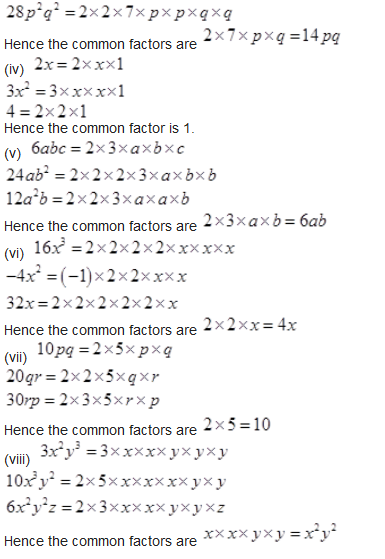Question 2.
Factorize the following expressions.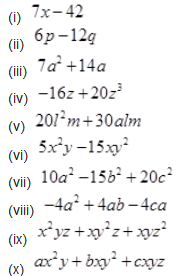Solution :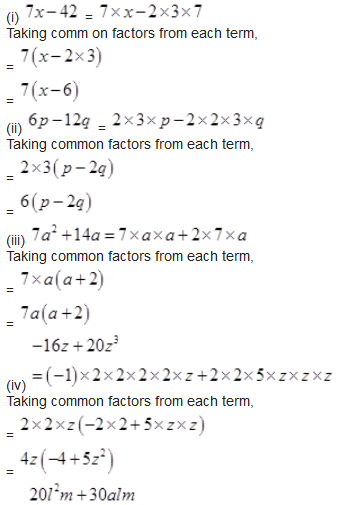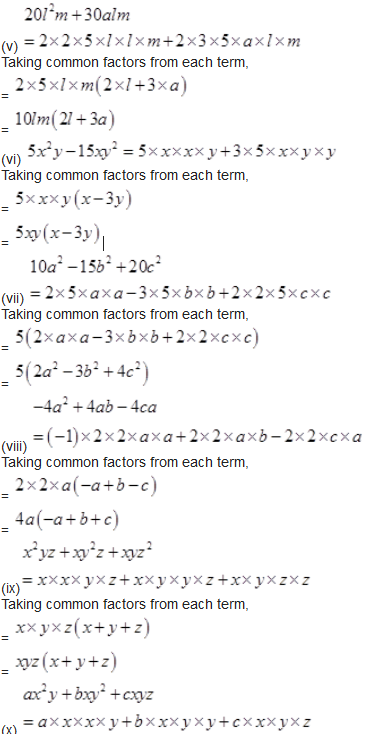Taking common factors from each term,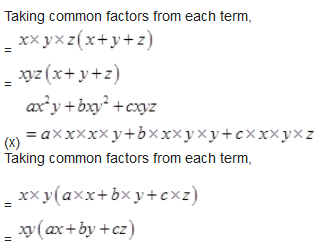Question 3.
Factorize: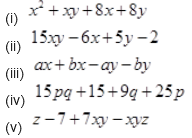Solution :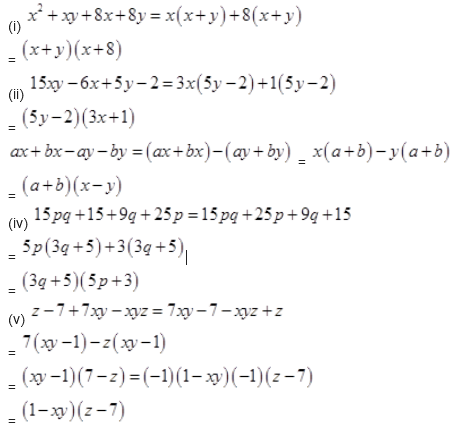NCERT Solutions for Class 8 Maths Exercise 14.2
Question 1.
Factorize the following expressions: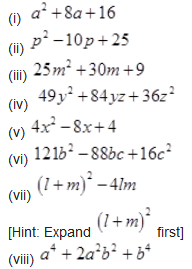Solution :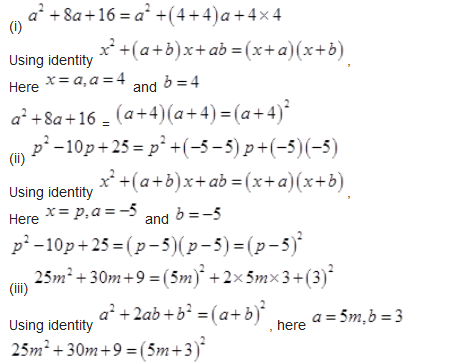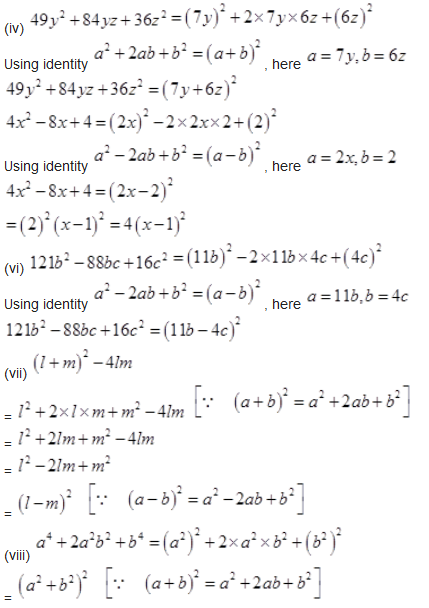Question 2.
Factorize
(i)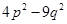(ii)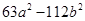(iii)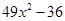(iv)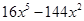(v)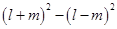(vi)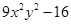(vii)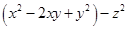(viii)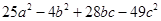Solution :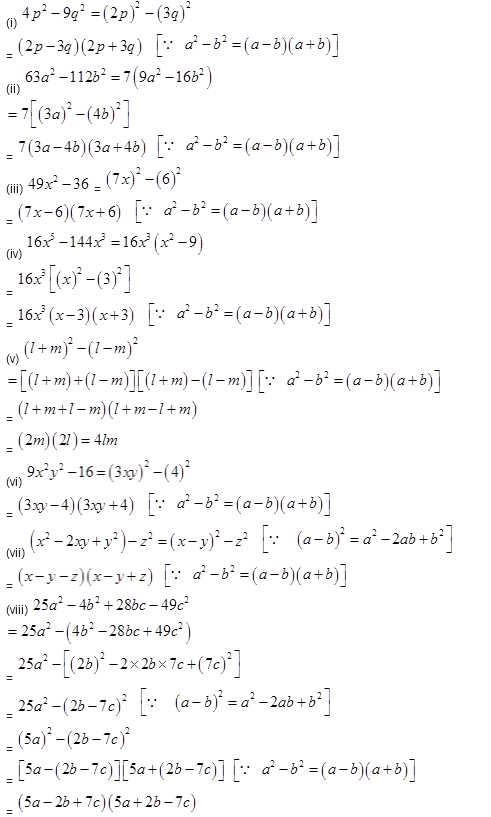Question 3.
Factorize the expressions: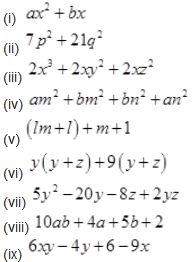Solution :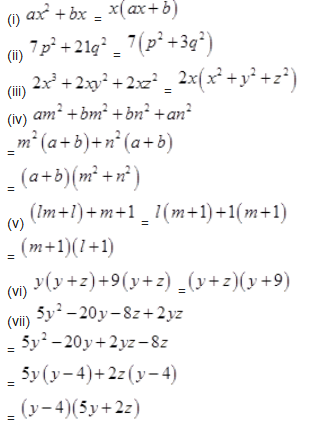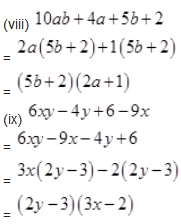Question 4.
Factorize: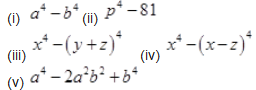Solution :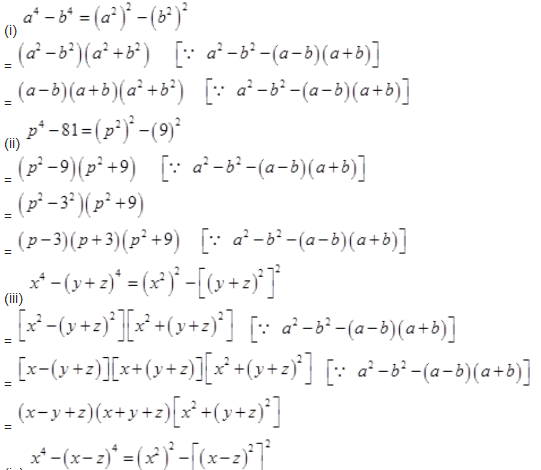(iv)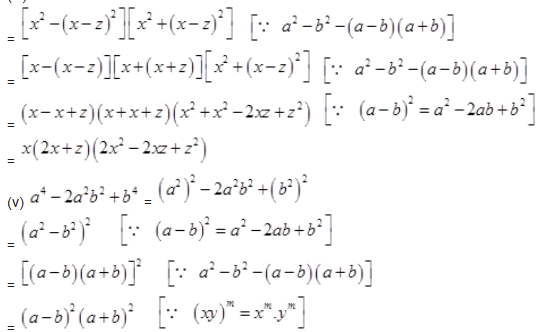Question 5.
Factorize the following expressions: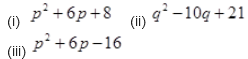Solution :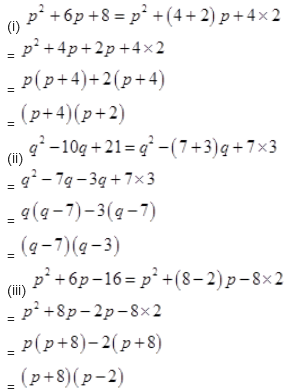NCERT Solutions for Class 8 Maths Exercise 14.3
Question 1.
Carry out the following divisions: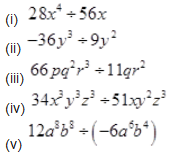Solution :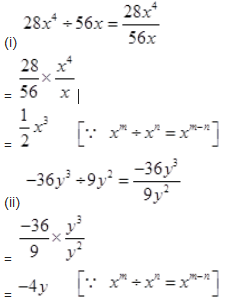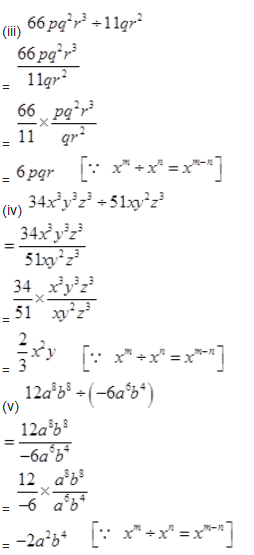Question 2.
Divide the given polynomial by the given monomial: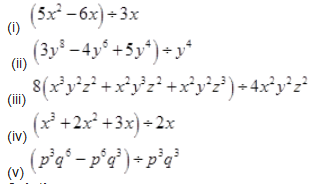Solution :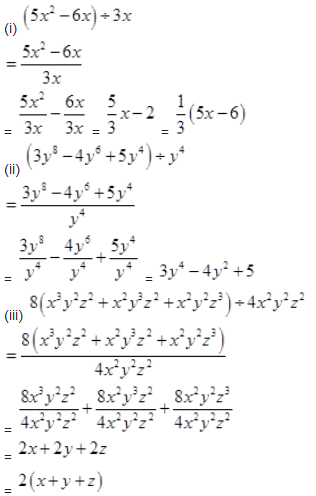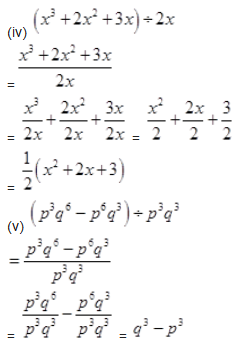Question 3.
Work out the following divisions: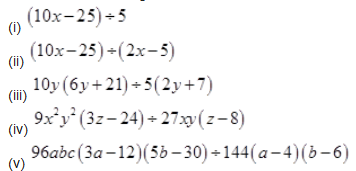Solution :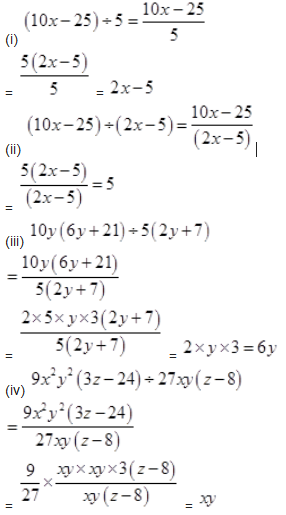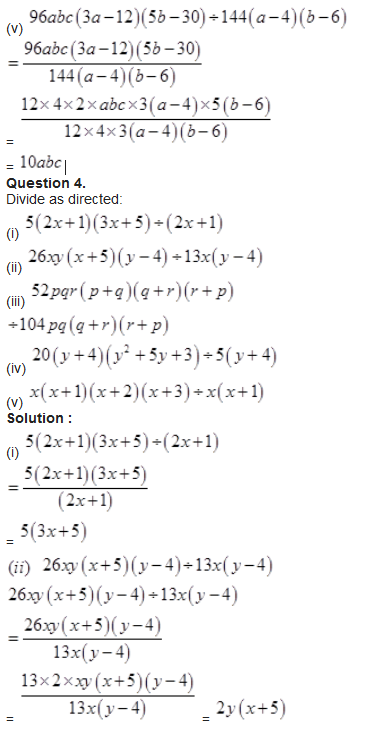(iii)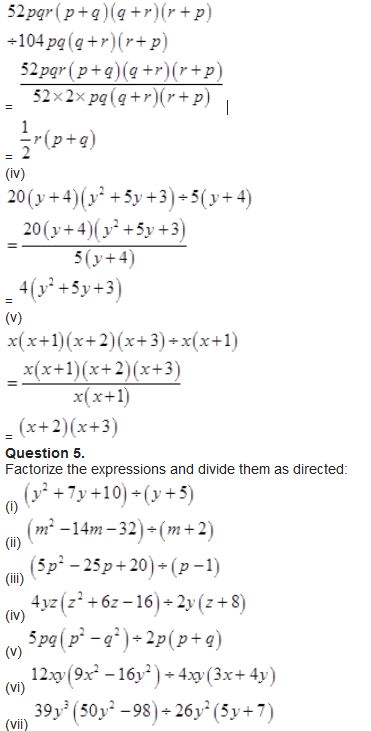Solution :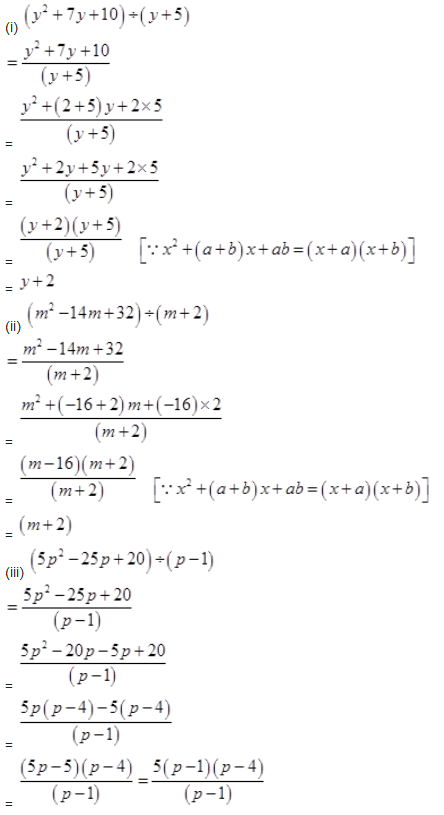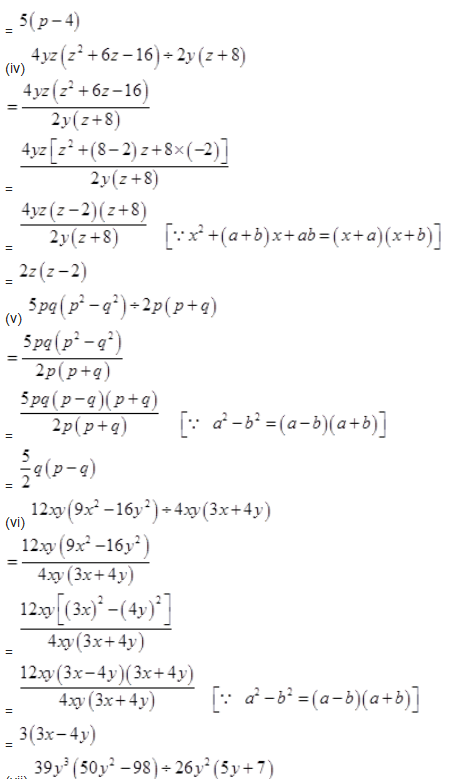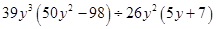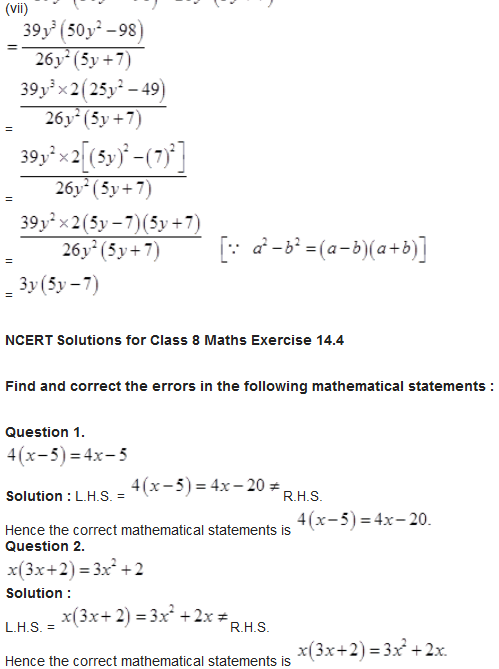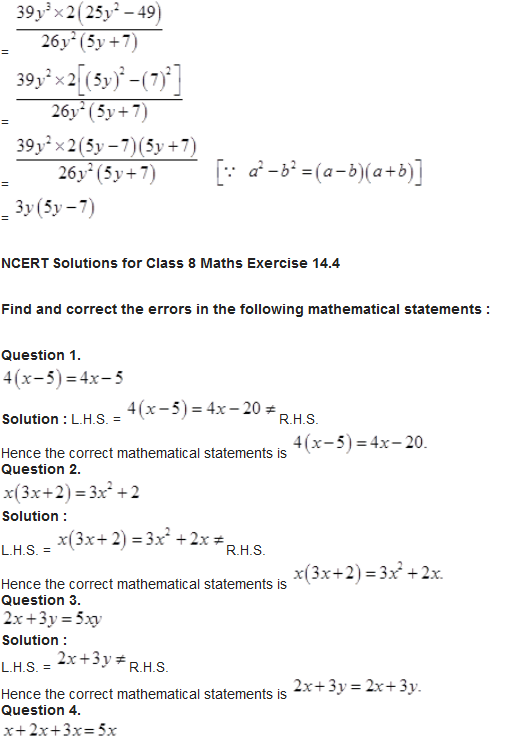Solution :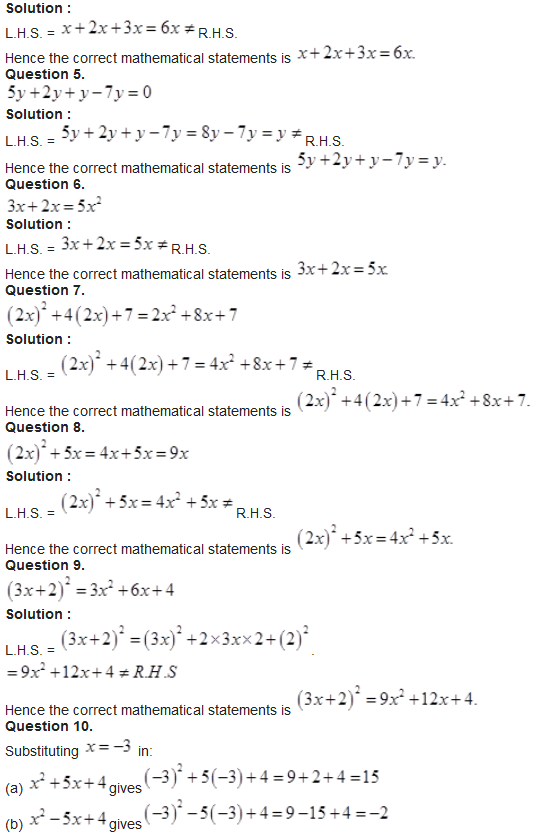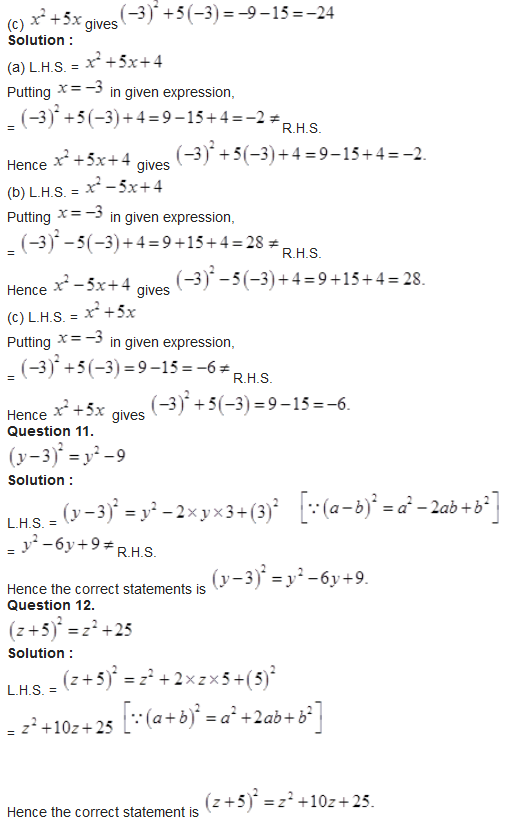Question 13.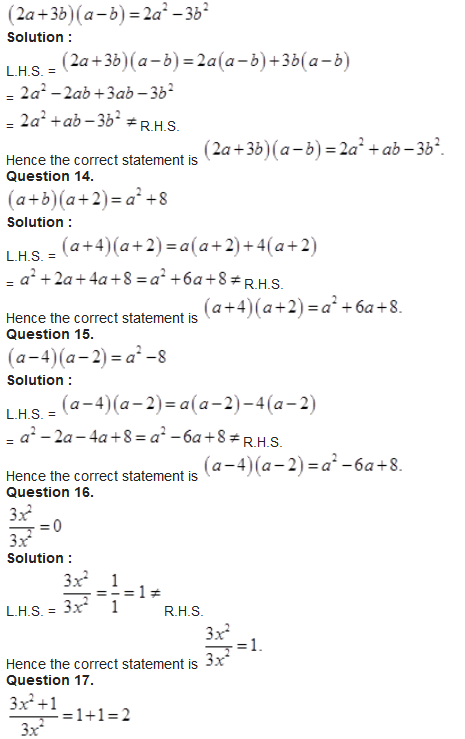Solution :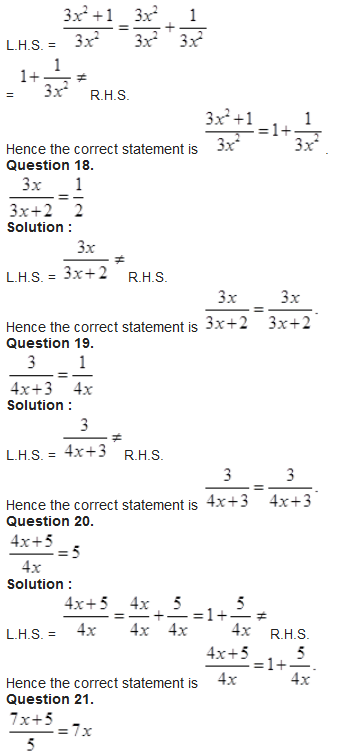Solution :
L.H.S. =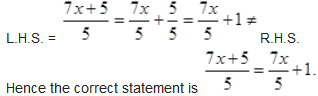R.H.S.

Get 30% off your first purchase!

X
error: Content is protected !!
Scroll to Top